STRONG AND WEAK ACIDS

```
```

This page explains the terms strong and weak as applied to acids. As a part of this it defines and explains what is meant by pH, Ka and pKa.

It is important that you don't confuse the words strong and weak with the terms concentrated and dilute.

As you will see below, the strength of an acid is related to the proportion of it which has reacted with water to produce ions. The concentration tells you about how much of the original acid is dissolved in the solution.

It is perfectly possible to have a concentrated solution of a weak acid, or a dilute solution of a strong acid. Read on . . .

Strong acids

Explaining the term "strong acid"

We are going to use the Bronsted-Lowry definition of an acid.

Note:  If you don't know what the Bronsted-Lowry theory of acids is, you should read about theories of acids and bases on another page in this section. You don't need to spend time reading about Lewis acids and bases for the purposes of this present page.

When an acid dissolves in water, a proton (hydrogen ion) is transferred to a water molecule to produce a hydroxonium ion and a negative ion depending on what acid you are starting from.

In the general case . . .These reactions are all reversible, but in some cases, the acid is so good at giving away hydrogen ions that we can think of the reaction as being one-way. The acid is virtually 100% ionised.

For example, when hydrogen chloride dissolves in water to make hydrochloric acid, so little of the reverse reaction happens that we can write: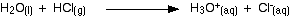At any one time, virtually 100% of the hydrogen chloride will have reacted to produce hydroxonium ions and chloride ions. Hydrogen chloride is described as a strong acid.

A strong acid is one which is virtually 100% ionised in solution.

Other common strong acids include sulphuric acid and nitric acid.

You may find the equation for the ionisation written in a simplified form: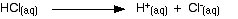This shows the hydrogen chloride dissolved in the water splitting to give hydrogen ions in solution and chloride ions in solution.

This version is often used in this work just to make things look easier. If you use it, remember that the water is actually involved, and that when you write H+(aq) what you really mean is a hydroxonium ion, H3O+.

Note:  You should find out what your examiners prefer on this. You are unlikely to find this from your syllabus, but should look at recent exam papers and mark schemes. If you are doing a UK-based exam and haven't got copies of your syllabus and past papers, you should have! Follow this link to find out how to get hold of them.

Strong acids and pH

pH is a measure of the concentration of hydrogen ions in a solution. Strong acids like hydrochloric acid at the sort of concentrations you normally use in the lab have a pH around 0 to 1. The lower the pH, the higher the concentration of hydrogen ions in the solution.

Defining pH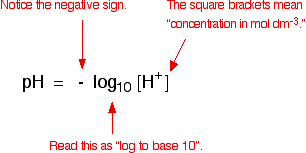Note:  If you are asked to define pH in an exam, simply write down the expression in black. Never try to define it in words - it is a waste of time, and you are too likely to miss something out (like mentioning that the concentration has to be in mol dm-3). In the expression, above, the square brackets imply that, so you don't need to mention it.

Working out the pH of a strong acid

Suppose you had to work out the pH of 0.1 mol dm-3 hydrochloric acid. All you have to do is work out the concentration of the hydrogen ions in the solution, and then use your calculator to convert it to a pH.

With strong acids this is easy.

Hydrochloric acid is a strong acid - virtually 100% ionised. Each mole of HCl reacts with the water to give 1 mole of hydrogen ions and 1 mole of chloride ions

That means that if the concentration of the acid is 0.1 mol dm-3, then the concentration of hydrogen ions is also 0.1 mol dm-3.

Use your calculator to convert this into pH. My calculator wants me to enter 0.1, and then press the "log" button. Yours might want you to do it in a different order. You need to find out!

log10 [0.1] = -1

But pH = - log10 [0.1]

- (-1) = 1

The pH of this acid is 1.

Note:  If you want more examples to look at and to try yourself (with fully worked solutions given), you may be interested in my chemistry calculations book. This also includes the slightly more confusing problem of converting pH back into hydrogen ion concentration.

Weak acids

Explaining the term "weak acid"

A weak acid is one which doesn't ionise fully when it is dissolved in water.

Ethanoic acid is a typical weak acid. It reacts with water to produce hydroxonium ions and ethanoate ions, but the back reaction is more successful than the forward one. The ions react very easily to reform the acid and the water.At any one time, only about 1% of the ethanoic acid molecules have converted into ions. The rest remain as simple ethanoic acid molecules.

Most organic acids are weak. Hydrogen fluoride (dissolving in water to produce hydrofluoric acid) is a weak inorganic acid that you may come across elsewhere.

Note:  If you are interested in exploring organic acids further, you will find them explained elsewhere on the site. It might be a good idea to read the rest of this page first, though.

If you want to know why hydrogen fluoride is a weak acid, you can find out by following this link.

These pages are in completely different parts of this site. If you follow either link, use the BACK button to return to this current page.

Comparing the strengths of weak acids

The position of equilibrium of the reaction between the acid and water varies from one weak acid to another. The further to the left it lies, the weaker the acid is.Note:  If you don't understand about position of equilibrium follow this link before you go any further.

You are also going to need to know about equilibrium constants, Kc for homogeneous equilibria. There is no point in reading any more of this page unless you do!

The acid dissociation constant, Ka

You can get a measure of the position of an equilibrium by writing an equilibrium constant for the reaction. The lower the value for the constant, the more the equilibrium lies to the left.

The dissociation (ionisation) of an acid is an example of a homogeneous reaction. Everything is present in the same phase - in this case, in solution in water. You can therefore write a simple expression for the equilibrium constant, Kc.

Here is the equilibrium again:You might expect the equilibrium constant to be written as: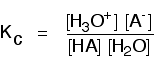At the bottom of the expression, you have a term for the concentration of the water in the solution. That's not a problem - except that the number is going to be very large compared with all the other numbers.

In 1 dm3 of solution, there are going to be about 55 moles of water.

Note:  1 mole of water weighs 18 g. 1 dm3 of solution contains approximately 1000 g of water. Divide 1000 by 18 to get approximately 55.

If you had a weak acid with a concentration of about 1 mol dm-3, and only about 1% of it reacted with the water, the number of moles of water is only going to fall by about 0.01. In other words, if the acid is weak the concentration of the water is virtually constant.

In that case, there isn't a lot of point in including it in the expression as if it were a variable. Instead, a new equilibrium constant is defined which leaves it out. This new equilibrium constant is called Ka.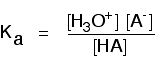You may find the Ka expression written differently if you work from the simplified version of the equilibrium reaction: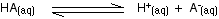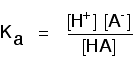This may be written with or without state symbols.

It is actually exactly the same as the previous expression for Ka! Remember that although we often write H+ for hydrogen ions in solution, what we are actually talking about are hydroxonium ions.

This second version of the Ka expression isn't as precise as the first one, but your examiners may well accept it. Find out!

```
```

To take a specific common example, the equilibrium for the dissociation of ethanoic acid is properly written as:The Ka expression is: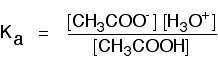```
```

If you are using the simpler version of the equilibrium . . .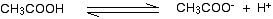. . . the Ka expression is: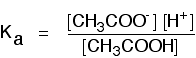Note:  Because you are likely to come across both of these versions depending on where you read about Ka, you would be wise to get used to using either. For exam purposes, though, use whichever your examiners seem to prefer.

The table shows some values of Ka for some simple acids:

acidKa (mol dm-3)
hydrofluoric acid5.6 x 10-4
methanoic acid1.6 x 10-4
ethanoic acid1.7 x 10-5
hydrogen sulphide8.9 x 10-8

These are all weak acids because the values for Ka are very small. They are listed in order of decreasing acid strength - the Ka values get smaller as you go down the table.

However, if you aren't very happy with numbers, that isn't immediately obvious. Because the numbers are in two parts, there is too much to think about quickly!

To avoid this, the numbers are often converted into a new, easier form, called pKa.

```
```

An introduction to pKa

pKa bears exactly the same relationship to Ka as pH does to the hydrogen ion concentration: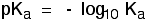If you use your calculator on all the Ka values in the table above and convert them into pKa values, you get:

acidKa (mol dm-3)pKa
hydrofluoric acid5.6 x 10-43.3
methanoic acid1.6 x 10-43.8
ethanoic acid1.7 x 10-54.8
hydrogen sulphide8.9 x 10-87.1

Note:  Notice that unlike Ka, pKa doesn't have any units.

Notice that the weaker the acid, the larger the value of pKa. It is now easy to see the trend towards weaker acids as you go down the table.

Remember this:

• The lower the value for pKa, the stronger the acid.

• The higher the value for pKa, the weaker the acid.

Note:  If you need to know about Ka and pKa, you are quite likely to need to be able to do calculations with them. You will probably need to be able to calculate the pH of a weak acid from its concentration and Ka or pKa. You may need to reverse this and calculate a value for pKa from pH and concentration. I can't help you with these calculations on this site, but they are all covered in detail in my chemistry calculations book.

```
```
 Questions to test your understanding If this is the first set of questions you have done, please read the introductory page before you start. You will need to use the BACK BUTTON on your browser to come back here afterwards. questions on strong and weak acids answers
```
```

Where would you like to go now?

To the acid-base equilibria menu . . .

To the Physical Chemistry menu . . .

To Main Menu . . .

```
```

© Jim Clark 2002 (modified November 2013)